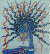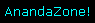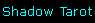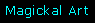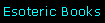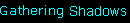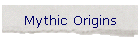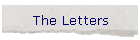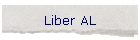© linda falorio, 1978, 1996, 1998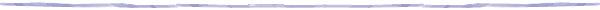Keys to the Magick of the Dark

 A =  1 B =  5 C =  9 D =  12 E =   2 F =   8 G =  10 H =  0 (2) I  =  3 J  =  6 K =  9 L  = 14 M =  6 N = 13 O = 4 P =  7 Q = 18 R = 15 S = 16 T = 11 U = 0 (5) V = 8 W = 0 (10) X = 11 Y = 6 Z = 32© AnandaZone 1998 - 2015 All contents © Linda Falorio unless otherwise noted. The English Qabalah™, EQ™, and E.Q.™ are trademarks of Anandazone anandazone@anandazone.nu Linda Falorio / Fred Fowler Pittsburgh, PA 15224 U S A